# Excel FV function to calculate future value

This tutorial looks at how to use the FV function in Excel to find the future value of a series of periodic payments and a single lump-sum payment.

Building your personal and corporate finances requires thorough planning. One of the most important factors of success is understanding how much an investment made today will grow to in the future. That is called the future value of investment, and this tutorial will teach you how to calculate it in Excel.

## Future value in Excel

The future value (FV) is one of the key metrics in financial planning that defines the value of a current asset in the future. In other words, FV measures how much a given amount of money will be worth at a specific time in the future.

Normally, the FV calculation is based on an anticipated growth rate, or rate of return. When the money is deposited in a saving account with a predefined interest rate, determining a future value is quite easy. The FV of investments in stocks, bonds or other securities may be hard to calculate accurately because of a volatile rate of return.

Luckily, Microsoft Excel provides a special function that does all the math behind the scenes based on the arguments that you specify.

## Excel FV function

FV is an Excel financial function that returns the future value of an investment based on a fixed interest rate. It works for both a series of periodic payments and a single lump-sum payment.

The function is available in all versions Excel 365, Excel 2019, Excel 2016, Excel 2013, Excel 2010 and Excel 2007.

The FV syntax is as follows:

FV(rate, nper, pmt, [pv], [type])

Where:

• Rate (required) - the interest rate per period. If you pay once a year, supply an annual interest rate; if you pay each month, then you should specify a monthly interest rate, and so on.
• Nper (required) - the total number of payment periods for the lifetime of an annuity.
• Pmt (optional) - the constant amount paid each period. Should be expressed as a negative number. If omitted, it is assumed to be 0, and the pv argument must be included.
• Pv (optional) - the present value of the investment. Should be represented by a negative number. If omitted, it defaults to 0, and the pmt argument must be included.
• Type (optional) - indicates when the payments are made:
• 0 or omitted (default) - at the end of a period (regular annuity)
• 1 - at the beginning of a period (annuity due)

### 4 things to remember about Excel FV function

To correctly build a FV formula in your worksheets and avoid common errors, please keep in mind these usage notes:

1. For any inflows such as dividends or other earnings, use positive numbers. For any outflows such as deposits to a saving or investing account, use negative numbers.
2. If the present value (pv) is zero or omitted, the payment amount (pmt) must be included, and vice versa.
3. The rate argument can be expressed as a percentage or decimal number, e.g. 8% or 0.08.
4. To get the correct future value, you must be consistent with nper and rate. For instance, if you make 3 yearly payments at an annual interest rate of 5%, use 3 for nper and 5% for rate. If you do a series of monthly investments for a period of 3 years, then use 3*12 (a total of 36 payments) for nper and 5%/12 for rate.

## Basic future value formula in Excel

This example shows how to use the FV function in Excel in its simplest form to calculate future value, given a periodic interest rate, the total number of periods, and a constant payment amount per period.

• Periodic interest rate (rate): C2
• Number of periods (nper): C3
• Payment amount (pmt): C4

Let's say you are going to make a yearly \$1,000 payment for 10 years with an annual interest rate of 6%. It is assumed to be a regular annuity where all payments are made at the end of the year.

To find the future value, configure the FV function in this way:

`=FV(C2, C3, C4)`

Please notice that pmt is a negative number because this money is paid out.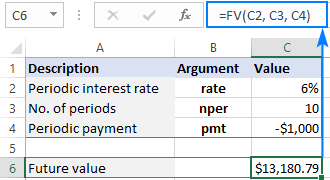If the payment is represented by a positive number, don't forget to put the minus sign right before the pmt argument:

`=FV(C2, C3, -C4)`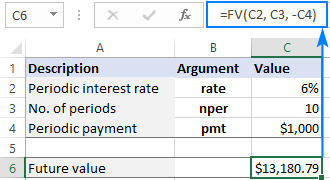## How to calculate future value in Excel - formula examples

The basic Excel FV formula is very simple, right? Now, let's have a look at how to tweak it to handle a couple of most common scenarios.

### FV formula for periodic payments

When investing money through a series of regular savings, it often happens that you are provided with an annual interest rate and the investment term defined in years, whereas the payments are to be made weekly, monthly, quarterly or semiannually. In such situations, it is very important that the rate and nper units be consistent.

To convert an annual interest rate to a periodic rate, divide the annual rate by the number of periods per year:

• Monthly payments: rate = annual interest rate / 12
• Quarterly payments: rate = annual interest rate / 4
• Semiannual payments: rate = annual interest rate / 2

To get the total number of periods, multiply the term in years by the number of periods per year:

• Monthly payments: nper = no. of years * 12
• Quarterly payments: nper = no. of years * 4
• Semiannual payments: nper = no. of years * 2

Now, let's see how it works in practice. Suppose you monthly invest \$200 for 3 years with an annual interest rate of 6%. The source data is input in these cells:

• Annual interest rate (B2): 6%
• No. of years (B3): 3
• Monthly payment (B4): -200
• Periods per year (B5): 12

To calculate the future value of this investment, the formula in B7 is:

`=FV(B2/B5, B3*B5, B4)`

As shown in the image below, the same formula determines the future value based on quarterly savings equally well: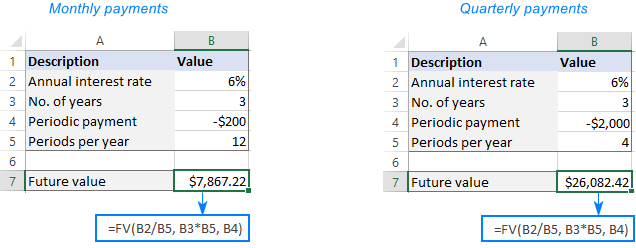### FV formula for lump-sum investment

If you choose to invest money as a one-time lump sum payment, the future value formula is based on the present value (pv) rather than periodic payment (pmt).

So, we set up our sample data as follows:

• Annual interest rate (C2): 7%
• No. of years (C3): 5
• Present value (C4): -1000

The formula to calculate the future value of the investment is:

`=FV(C2, C3, ,C4)`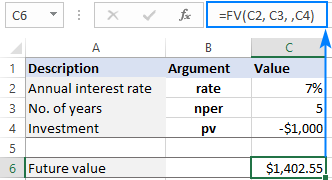• The investment amount (pv) is a negative number because it's an outflow.
• The pmt argument is 0 or omitted.

If the compounding periods for your investment are not annual, then to determine the future value accurately, you need to make the following adjustments to the formula:

• For rate, divide an annual interest rate by the number of compounding periods per year.
• For nper, multiply the number of years by the number of compounding periods per year.

As an example, let's find the future value of the above investment with an interest rate compounded monthly. For this, we divide an annual interest rate (C2) by 12 and multiply the number of years (C3) by 12:

`=FV(C2/12, C3*12, ,C4)`

or

`=FV(C2/C5, C3*C5, ,C4)`

Where C5 is the number of compounding periods per year:### Get future value for different compounding periods

To compare the amount of growth generated by various compounding periods, you need to supply different rate and nper to the FV function.

To have all calculations performed with a single formula, do the following:

• Input the number of compounding periods per year in B2.
• Arrange your data like shown in the image below.
• Enter the following formula in C2 and drag it down through C6:
`=FV(\$F\$2/B2, \$F\$3*B2, ,\$F\$4)`

Please pay attention that we lock the annual interest rate (\$F\$2), the number of years (\$F\$3) and the investment amount (\$F\$4) references with the dollar sign (\$) so they won't shift when copying down the formula.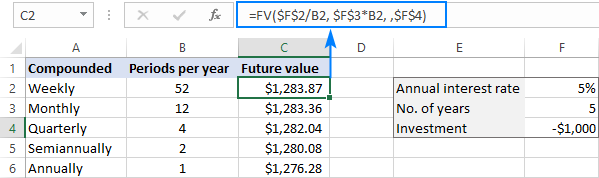## Make a future value calculator in Excel

If your goal is to build a universal FV calculator that works for both periodic and lump-sum payments with either annuity type, then you will need to use the Excel FV function in its full form.

For starters, allocate cells for all the arguments, including the optional ones like shown in the screenshot below. And then, define the arguments in this way:

• Rate (periodic interest rate): B2/ B7 (annual interest rate / periods per year)
• Nper (total number of payment periods): B3*B7 (number of years * periods per year)
• Pmt (periodic payment amount): B4
• Pv (initial investment): B5
• Type (when payments are due): B6
• Compounding periods per year: B7

Putting the arguments together, we get this formula:

`=FV(B2/B7, B3*B7, B4, B5, B7)`

Suppose you wish to save some money for renovating your house in 5 years. You deposit \$3,000 to your saving account at an interest rate of 7% compounded monthly. Furthermore, you are going to add \$100 at the beginning of each month. How much money will there be in your saving account in 5 years? According to our Excel FV calculator - around \$11,500.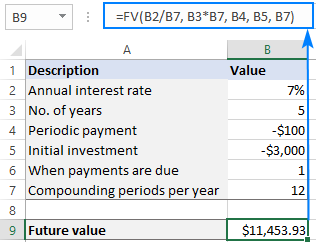When setting up a future value calculator for other users, there are a few things to take notice of:

• Both pmt and pv should be negative numbers because they represent an outflow. If positive numbers are entered in the corresponding cells, then put the minus sign before these arguments directly in the formula.
• If pmt is zero or omitted, be sure to specify the present value (pv) and vice versa.
• For type, consider creating a drop-down list to only allow 0 and 1 values. This will help you prevent accidental mistakes that users could make.
• The Compounding periods per year cell (B7) must have a number in it other than zero, otherwise the formula will return a #DIV/0 error. If an interest rate is compounded annually, enter 1 in that cell.

## Excel FV function not working

If a FV formula results in an error or yields a wrong result, in all likelihood, that will be one of the following.

### #VALUE! error

May occur if one or more arguments are non-numeric. To fix the error, check if any of the numbers referenced in your formula are formatted as text. If some are, then convert text values to numbers.

### FV function returns an incorrect future value

If the returned future value is negative or much lower than expected, most likely, either the pmt or pv argument, or both, are represented by positive numbers. Please remember that negative numbers should be used for all outgoing payments.

That's how to how to calculate future value of annuity in Excel. I thank you for reading and hope to see you on our blog next week!